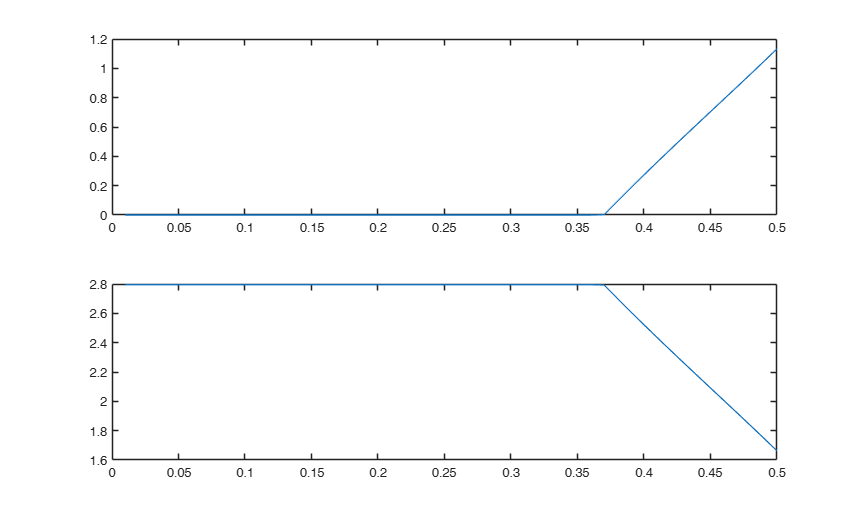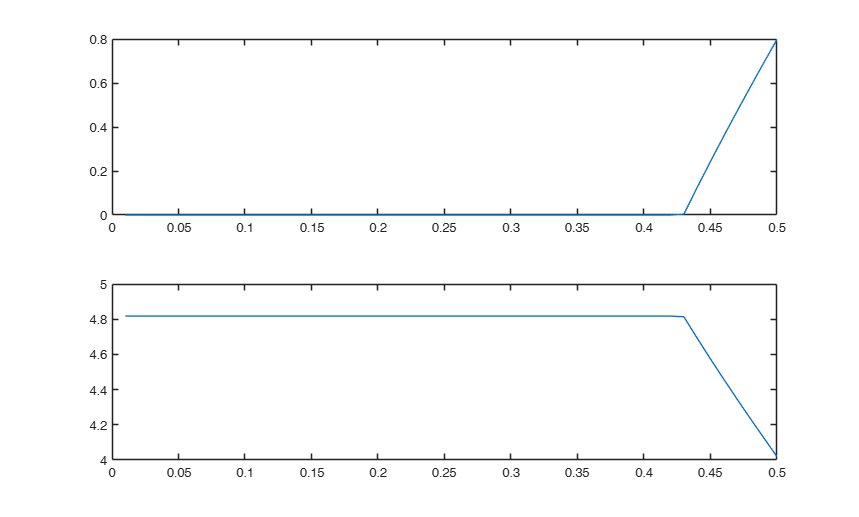# RKbdp

RKbdp finds the constants c associated to the supplied breakdown point and asymptotic rejection point

## Syntax

• c=RKbdp(bdp,v)example
• c=RKbdp(bdp,v,ARP)example
• [c,M]=RKbdp(___)example

## Description

 c =RKbdp(bdp, v) Find c and M given bdp and ARP.

 c =RKbdp(bdp, v, ARP) Computation of c and M for a series of values of bdp (v=3).

 [c, M] =RKbdp(___) Computation of c and M for a series of values of bdp (v=10).

## Examples

expand all

### Find c and M given bdp and ARP.

bdp=0.5;
% Suppose the dimension is 3
v=3;
% The constants c and M associated to a breakdown point of 50 per cent
% and an ARP of 0.05 when there are three variables are
% c=1.133183024897769 and M= 1.662300458017338
[c,M]=RKbdp(bdp,v)

###Computation of c and M for a series of values of bdp (v=3).

v=3;
bdp=0.01:0.01:0.5;
cM=zeros(length(bdp),2);
for i=1:length(bdp)
[c,M]=RKbdp(bdp(i),v);
cM(i,:)=[c M];
end
subplot(2,1,1)
plot(bdp,cM(:,1))
subplot(2,1,2)
plot(bdp,cM(:,2))###Computation of c and M for a series of values of bdp (v=10).

ARP fixed to 0.01.

v=10;
bdp=0.01:0.01:0.5;
cM=zeros(length(bdp),2);
for i=1:length(bdp)
[c,M]=RKbdp(bdp(i),v,0.01);
cM(i,:)=[c M];
end
subplot(2,1,1)
plot(bdp,cM(:,1))
subplot(2,1,2)
plot(bdp,cM(:,2))## Input Arguments

### bdp — breakdown point. Scalar.

Scalar defining breakdown point (i.e a number in the interval [0 0.5). Please notice that the maximum achievable breakdown point is (n-p)/(2*n), and therefore the value 0.5 is reached only when the sample size goes to infinity. However, this routine assume a sample of size infinity and allows you to specify a bdp equal to 0.5.

Data Types: single|double

### v — number of response variables. Scalar.

e.g. in regression v=1

Data Types: single|double|int32|int64

### ARP — asymptotic rejection probability. Scalar.

The asymptotic rejection probability of an estimator is defined as the probability in large sample under a reference distribution that a Malanobis distance excees $c_0$, where $c_0=inf \{ u_0 | w(u)=0, \forall u>u_0 \}$.

$w(u)$ is the weight function (defined in RKwei.m). In other words, given $c_0=sup(\rho(u))$,if an estimator is normed to the normal distribution ARP is $1-F_{\chi^2_v}(c_0^2)$.

The default value of ARP is 0.05.

Example: 0.04 

Data Types: double

## Output Arguments

### c —Requested tuning constant. Scalar

Tuning constatnt of Rocke rho function (translated Tukey Biweight) associated to requested breakdown point and asymptotic rejection probability

### M —Requested tuning constant. Scalar

Tuning constant of Rocke rho function (translated Tukey Biweight) associated to requested breakdown point and asymptotic rejection probability

Maronna, R.A., Martin D. and Yohai V.J. (2006), "Robust Statistics, Theory and Methods", Wiley, New York.

Rocke D.M. (1996), Robustness properties of S-estimators of multivariate location and shape in high dimension, The Annals of Statistics, Vol. 24, pp. 1327-1345.